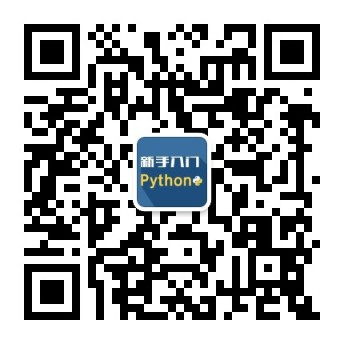2016
09-20

# PHP7标量类型声明

• 强制方式- 强制性是默认模式，不需要指定

• 严格方式 - 严格模式有明确的暗示

• int
• float
• bool
• string
• interfaces
• array
• callable

## 示例 - 强制模式

```<?php
// Coercive mode
function sum(int ...\$ints) {
return array_sum(\$ints);
}
print(sum(2, '3', 4.1));
?>
```

```9
```

## 示例 - 严格模式

```<?php
// Strict mode
declare(strict_types=1);
function sum(int ...\$ints) {
return array_sum(\$ints);
}
print(sum(2, '3', 4.1));
?>
```

`Fatal error: Uncaught TypeError: Argument 2 passed to sum() must be of the type integer, string given, ...`